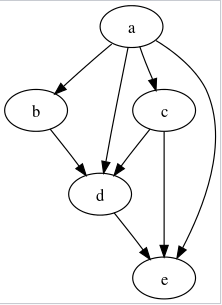Problem title
Difficulty
Avg time to solve

Program for Priority CPU Scheduling | Set 1
Easy
15 mins
Online Majority Element In Subarray
Moderate
15 mins
Create Sorted Array through Instructions
Moderate
20 mins
Lexicographically smallest equivalent string
Moderate
25 mins
Block Heights
Easy
20 mins
Identical sentences
Hard
40 mins
Find All Paths In DAG
Moderate
30 mins
Max Town Order
Easy
15 mins
Second Minimum Node In A Binary Tree
Easy
20 mins
Find The Sum Of The Left Leaves
Moderate
25 mins3

# Find All Paths In DAG

Difficulty: MEDIUM
Avg. time to solve
30 min
Success Rate
70%

Problem Statement

#### You are supposed to find all possible paths from node 0 to node n - 1.

##### Note
``````Nodes are numbered from 0 to N - 1.

The graph given is connected.

Print the path[][] in sorted order i.e path[i] is an array containing the path from 0 to n-1, and path[i] is lexicographically smaller than path[i + 1] then path[i] should appear before path[i + 1].

Assume we have two solutions
S1: A1 B1 C1 D1
S2: A2 B2 C2 D2

S1 is lexicographically smaller than S2 iff,
A1 < A2 OR
A1 = A2 AND B1 < B2 OR
A1 = A2 AND B1 = B2 AND C1 < C2 OR
A1 = A2 AND B1 = B2 AND C1 = C2 AND D1 < D2
``````
##### For Example :
``````The following is an example of DAG i.e a directed graph with no cycles in it.
``````##### Input Format :
``````The first line contains a single integer ‘T’ denoting the number of test cases. The test cases are as follows.

The first line of each test case contains two integers separated by a single space,‘N’ and ‘M’, where ‘N’  denotes the number of the vertices and ‘M’ denotes the number of edges in the graph.

The next ‘M’ lines of each test case contain two integers ‘X’ and ‘Y’ separated by a single space, here ‘X’ and ’Y’ are the vertices connected by a directed edge from ‘X’ to ‘Y’.
``````
##### Output Format :
``````For each test case, print all possible paths from node 0 to N - 1 in sorted order.

Print the output of each test case on a new line.
``````
##### Note :
``````You don’t need to print anything; It has already been taken care of.
``````
##### Constraints :
``````1 <= T <= 5
2 <= N <= 16
N-1 <= M <= N*(N - 1)/2
0 <= X,Y <= N - 1

Time Limit: 1 sec
``````
##### Sample Input 1 :
``````2
6 5
0 1
0 2
2 5
3 4
4 2
5 6
0 1
2 1
1 4
2 4
0 4
3 1
``````
##### Sample Output 1 :
``````0 2 5
0 1 4
0 4
``````
##### Explanation For Sample Output 1:
``````For the first test case,
All possible paths from 0 to 5 are:
0 -> 2 -> 5

For the second test case,
All possible paths from 0 to 5 are:
0 -> 1 -> 4
0 -> 4
As the first path appears before so it is printed first and then the second path.
``````

#### Sample Input 2:

``````2
4 4
0 1
0 2
0 3
1 2
2 1
0 1
``````
##### Sample Output 2:
``````0 3
0 1
``````Console